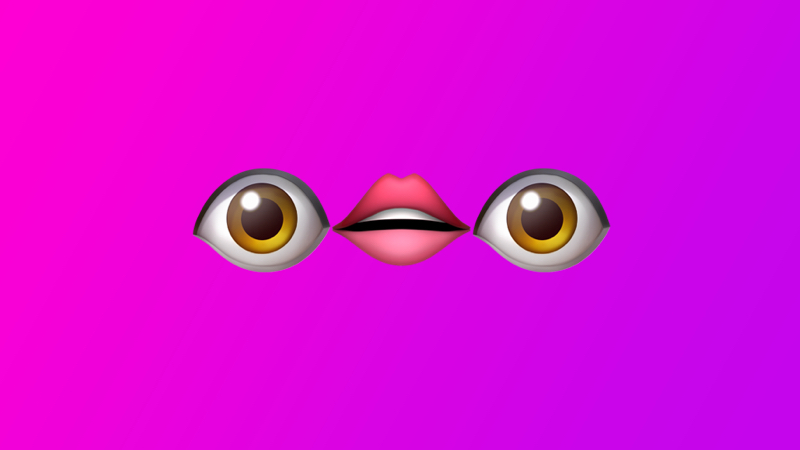# Why you should use strict equality 99% of the time

## and why is JavaScript so weird?

### Introduction

As we all know, JavaScript is a dynamically typed language.

Dynamically typed languages checks the type of a variable during run-time instead of compile-time.

In layman's terms, when you declare a value, JavaScript automatically converts its type without having to specify it explicitly.

This process is known as type coercion.

However, this ease of convenience produces unintended results.

#### Type Coercion

Type coercion is the automatic or implicit conversion of values from one data type to another (such as strings to numbers).

### Apples and OrangesWhat if I told you that Justin Timberlake's hair and instant noodles are not the same? It looks the same (disclaimer: may even smell or taste the same) but are totally different. Because they look the same, we assumed that they are the same.

This is what JavaScript's equality operator (double equals) does. It attempts to convert and compare operands of different types.

``````5 == 5 // is true as both are integers
"5" == 5 // is true due to type coercion

var a = "5" + 5 // integer 5 coerced to string
55 == a // true! wtfbbq???
"55" == a // also true! wtfbbq???

console.log(typeof a) // string``````

In this example, JavaScript coerced the integer 5 to a string type. However, the equality operator attempts to convert the type, thus returning true for both comparisons.

Let's look at another example.

``````false == 0 // is true because false coerces to 0
var a = false // set a to false boolean value
a == 0 // true but should be false``````

The comparison between the variable a and 0 returns true even though it is a boolean and not an integer.

What if we used explicit type coercion (aka type casting)?

``````var a = String(5)
console.log(typeof a) // string

var b = Number(5)
console.log(typeof b) // number

a == b // true``````

Again, the above example can cause all sorts of problems with your code.

It makes some sense as JavaScript is attempting to convert the values to the same type. The flipside is this makes it difficult to debug and anticipate what your code is doing.

### Not My Type

Q: What's the difference between the strict equality (triple equals ===) vs the equality (double equals ==) operator?

A: The strict equality operator saves lives (and relationships).#### Strict equality (===)

The strict equality operator (===) checks whether its two operands are equal, returning a Boolean result. Unlike the equality operator, the strict equality operator always considers operands of different types to be different. - Source

Let's try the examples again using the strict equality operator.

``````5 === 5 // is true as both are integers
"5" === 5 // false as they are different types

var a = "5" + 5 // integer 5 coerced to string
55 === a // false
"55" === a // true

console.log(typeof a) // string``````

``````false === 0 // false. One is a boolean, the other is an integer
var a = false // set a to false boolean value
a === 0 // false``````

How does this work in a real life scenario? Suppose you have a conditional statement that takes action depending on the comparison result.

Depending on which comparison operator that you use, it will return vastly different and possibly detrimental outcomes.

``````var a = 5
var b = "5"

a == b ? true : false // true
a === b ? true : false // false``````What the equality operator brings you.

### Conclusion

Due to this weird JavaScript quirk, you should start using the strict equality operator... like right now. It is going to save you a lot of heartaches and sleepness nights.

If all else fails, there's always TypeScript.